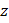# If a complex numberlies in the interior or on the boundary of a circle or radius 3 and centre atthen the greatest and least values ofare a)b)c)d) None of these

## Question ID - 103227 :- If a complex numberlies in the interior or on the boundary of a circle or radius 3 and centre atthen the greatest and least values ofare a)b)c)d) None of these

3537

(c)

It is given thatHence, the greatest value ofis 6

Since the least value of the modulus of a complex number is zerois satisfied byTherefore, the least value ofis 0

ALITER Here, we have to find the greatest and least of distances of all points lying inside or the circle from the pointIt is evident from the Fig. S.3, that the greatest distance is 6 whencoincides withand the least distance is 0 whencoindies withNext Question :
 If Im, then locus ofis a) An ellipse b) A parabola c) A straight line d) A circle